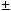# Electronics - Resistance and Power

### Exercise :: Resistance and Power - True or False

16.

An ohmmeter uses an internal battery to measure the resistance of a resistor.

 A. True B. False

Explanation:

No answer description available for this question. Let us discuss.

17.

A protoboard is used to make experimental circuits.

 A. True B. False

Explanation:

No answer description available for this question. Let us discuss.

18.

One horsepower is 746 watts.

 A. True B. False

Explanation:

No answer description available for this question. Let us discuss.

19.

Fixed-value resistors can internally short-circuit.

 A. True B. False

Explanation:

No answer description available for this question. Let us discuss.

20.

A resistor with a value of 9.9 k10% could have a value between 8.91 kand 10.89 k.

 A. True B. False